• ## python的矩阵乘法

千次阅读 2018-05-22 14:41:52
1 、对应元素相乘 element-wise product: np.multiply(), 或 *对应元素相乘如果不是相同规格的矩阵，这样就...2、同线性代数中矩阵乘法的定义： np.dot()np.dot(A, B)：对于二维矩阵，计算真正意义上的矩阵乘积，同...
1 、对应元素相乘 :  *对应元素相乘如果不是相同规格的矩阵，这样就有可能不能广播，比如3x1和2x1相乘就会报错，3x1和2x2相乘也会报错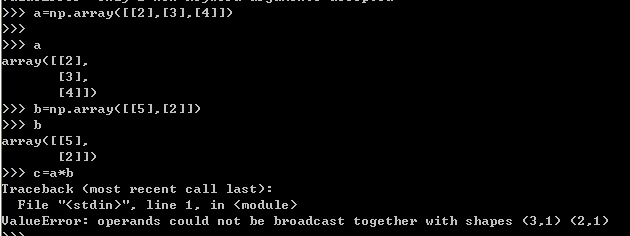所以要想使用该乘法，行和列要相同，或者a的列和b的行相同。2、同线性代数中矩阵乘法的定义： np.dot()np.dot(A, B)：对于二维矩阵，计算真正意义上的矩阵乘积，同线性代数中矩阵乘法的定义。对于一维矩阵，计算两者的内积。就是A矩阵的列要和B矩阵的行相同才可以进行计算｛mxn和nxp｝。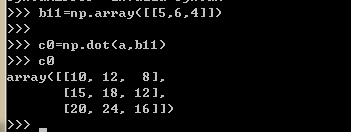3、一个是对应元素乘，一个是矩阵乘积3x1和1x2的两种乘法*和np.dot结果一样：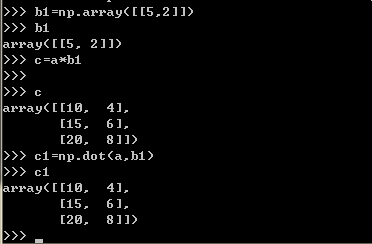2x2和2x2的两种乘法*和np.dot结果不一样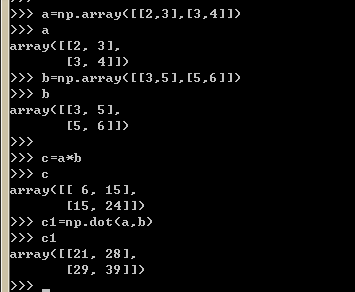展开全文• ## [Python]矩阵乘法

千次阅读 2018-02-02 13:38:42
np.dot(A, B)：对于二维矩阵，计算真正意义上的矩阵乘积，同线性代数中矩阵乘法的定义。对于一维矩阵，计算两者的内积。见如下Python代码： import numpy as np # 2-D array: 2 x 3 two_dim_matrix_one = np.array...

1. 同线性代数中矩阵乘法的定义： np.dot()

np.dot(A, B)：对于二维矩阵，计算真正意义上的矩阵乘积，同线性代数中矩阵乘法的定义。对于一维矩阵，计算两者的内积。见如下Python代码：
import numpy as np

# 2-D array: 2 x 3
two_dim_matrix_one = np.array([[1, 2, 3], [4, 5, 6]])
# 2-D array: 3 x 2
two_dim_matrix_two = np.array([[1, 2], [3, 4], [5, 6]])

two_multi_res = np.dot(two_dim_matrix_one, two_dim_matrix_two)
print('two_multi_res: %s' %(two_multi_res))

# 1-D array
one_dim_vec_one = np.array([1, 2, 3])
one_dim_vec_two = np.array([4, 5, 6])
one_result_res = np.dot(one_dim_vec_one, one_dim_vec_two)
print('one_result_res: %s' %(one_result_res))
12345678910111213141516
结果如下：
two_multi_res: [[22 28]
[49 64]]
one_result_res: 32123
2. 对应元素相乘 element-wise product: np.multiply(), 或 *

在Python中，实现对应元素相乘，有2种方式，一个是np.multiply()，另外一个是*。见如下Python代码：
import numpy as np

# 2-D array: 2 x 3
two_dim_matrix_one = np.array([[1, 2, 3], [4, 5, 6]])
another_two_dim_matrix_one = np.array([[7, 8, 9], [4, 7, 1]])

# 对应元素相乘 element-wise product
element_wise = two_dim_matrix_one * another_two_dim_matrix_one
print('element wise product: %s' %(element_wise))

# 对应元素相乘 element-wise product
element_wise_2 = np.multiply(two_dim_matrix_one, another_two_dim_matrix_one)
print('element wise product: %s' % (element_wise_2))12345678910111213
结果如下：
element wise product: [[ 7 16 27]
[16 35  6]]
element wise product: [[ 7 16 27]
[16 35  6]]

展开全文• 最近在熟悉python的...1、矩阵乘法 np.dot(a,b)，但a,b都为一维矩阵的时候，.dot实现内积，不用考虑a,b具体是行向量还是列向量，也就是说,a,b同为行向量仍然可以计算 a.dot(b) a@b 2、各个元素相乘 a*b ...
最近在熟悉python的科学计算，对于numpy的矩阵运算进行一些总结，和matlab还是很不一样的

import numpy as np

1、矩阵乘法

np.dot(a,b)，但a,b都为一维矩阵的时候，.dot实现内积，不用考虑a,b具体是行向量还是列向量，也就是说,a,b同为行向量仍然可以计算

a.dot(b)

a@b

2、各个元素相乘

a*b                       但当a,b分别为行向量和列向量时，返回矩阵，不管a,b谁在前边都是一样的结果

np.multiply(a,b)，但当a,b分别为行向量和列向量时，.multiply返回矩阵，不管a,b谁在前边都是一样的结果

3、行向量变列向量

a = np.array([1,2,3])

b=np.transpose([a])         修改b，不会对a进行修改

b = np.array([a]).T            修改b，不会对a进行修改

b = a.reshape(-1,1)          修改b，同时会对a进行修改

b = a[:,np.newaxis]           修改b，同时会对a进行修改

b=a.reshape(len(a),-1),    修改b，同时会对a进行修改

b=a[:,None].                     修改b，同时会对a进行修改

3.1、列向量变行向量

a = np.array([,,])

b = np.transpose(a)         修改b，会对a进行修改

4、列向量b乘以行向量a

np.multiply(a,b)

np.multiply(b,a)

a*b

b*a

np.outer(a,b)

np.outer(b,a)

b@a会报错

5、行向量a乘以列向量b

a@b

6、需要注意

对矩阵或向量a：a指的是第0行，

所以，对列向量a可以用a,a来表示第0,1个元素，但是对行向量a必须用a[:,n]来表示第n个元素，如果对a进行赋值，则是对整个行向量赋值。

参考：http://www.itkeyword.com/doc/7498360611061724524/numpy-scalar-multiplication-of-column-vector-times-row-vector

https://docs.scipy.org/doc/numpy/user/quickstart.html

https://www.cnblogs.com/bob-dong/p/6207871.html
展开全文numpy
• Python代码： import numpy as np if __name__ == '__main__': a = np.array([[2, -5, 0], [-1, 3, -4], [6, -8, -7], [-3, 0, 9]]) b = np.array([[4, -6], [7, 1], [3, 2]]) print(np.dot(a, b)...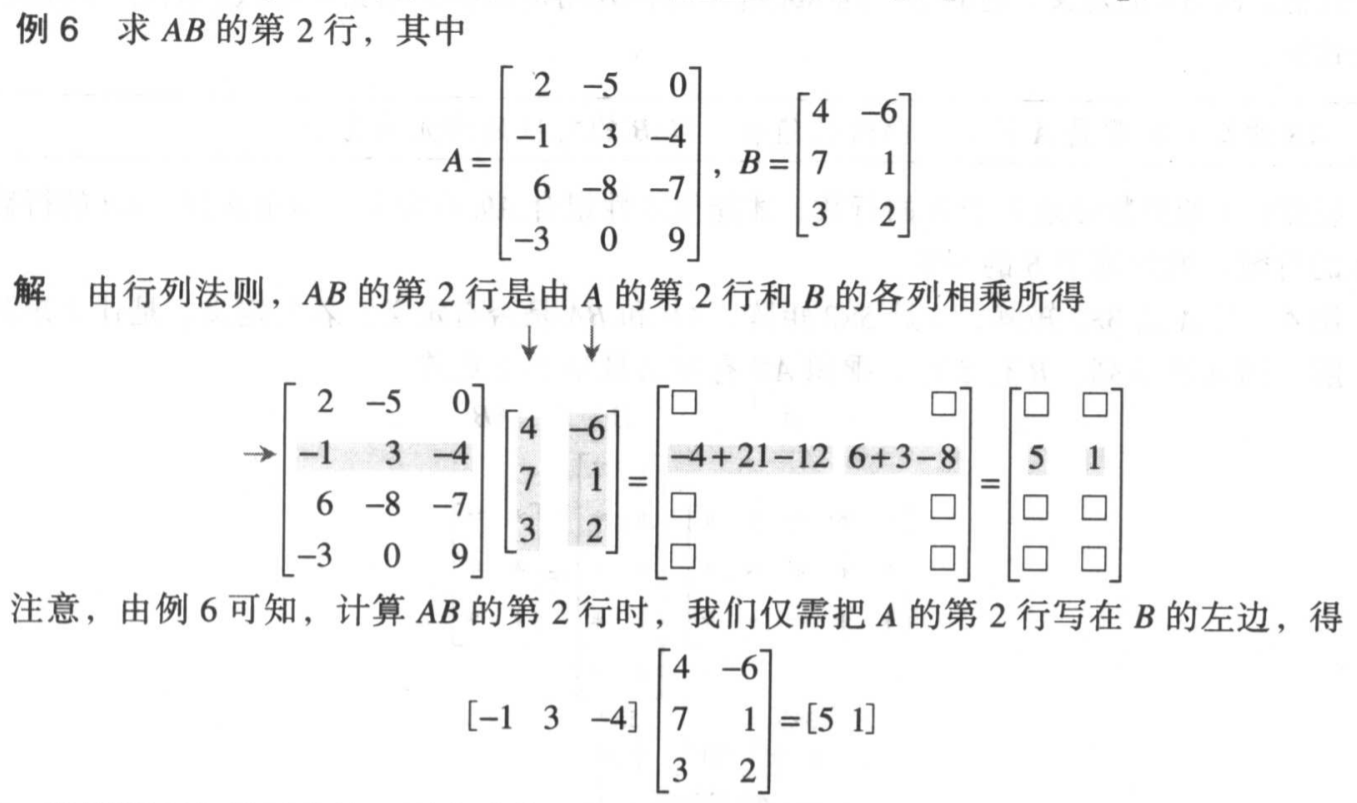Python代码：

import numpy as np

if __name__ == '__main__':
a = np.array([[2, -5, 0], [-1, 3, -4], [6, -8, -7], [-3, 0, 9]])
b = np.array([[4, -6], [7, 1], [3, 2]])

print(np.dot(a, b))

# 或者
print(np.matmul(a, b))


输出：

[[-27 -17]
[  5   1]
[-53 -58]
[ 15  36]]
[[-27 -17]
[  5   1]
[-53 -58]
[ 15  36]]


展开全文数学 线性代数
• python矩阵乘法，可以通过 NumPy 的np.dot()函数计算（乘积也称为点积）。 np.dot()接收两个NumPy数组作为参数，并返回数组的乘积。类似于数学中的两个矩阵相乘，A中每行的元素值与B中每列的元素值对应相乘再求和...
• 多线程计算矩阵乘法性能测试 目录： 1.手工方法计算乘法矩阵 1.1任意的 m**n 阶矩阵A和nk阶矩阵B核心代码（附件1） 1.2任意的两个m阶矩阵乘法用于测试代码运行效率（附件2） 2.分治法计算乘法矩阵 2.1普通递归分治法...java 多线程 算法 多进程
• 设计一个Python函数，计算两个矩阵（二维列表）的乘积。 函数接口定义 def multiply(a,b,p,q,r) a是一个p行q列的二维列表；b是一个q行r列的二维列表； 应返回矩阵p行r列的结果矩阵。 判断测试样例： p = int(input...
• 第三章，矩阵，02-python矩阵乘法1. 同线性代数中矩阵乘法的定义： np.dot()2. 对应元素相乘 element-wise product: np.multiply(), 或 * 搬运：Python中的几种矩阵乘法 1. 同线性代数中矩阵乘法的定义： np.dot() ...
• # TODO 计算矩阵乘法 AB，如果无法相乘则raise ValueError def matxMultiply(A, B): multiply = [] if len(A) != len(B): raise ValueError result = [list(row) for row in zip(*B)] for Al ...
• # TODO 计算矩阵乘法 AB，如果无法相乘则raise ValueError def matxMultiply(A, B): multiply = [] if len(A) != len(B): raise ValueError result = [list(row) for row in zip(*B)] for Al in ...
• Python中的矩阵乘法与线性代数中的矩阵乘法定义相同：np.dot()线性代数举例：Python代码举例对应元素相乘(element-wise product): np.multiply() 或 *线性代数举例：Python代码举例： 与线性代数中的矩阵乘法定义...numpy
• 内容包括矩阵操作（创建矩阵、矩阵加减法）、矩阵乘法、矩阵转置（求转置矩阵）、求方阵的迹、方阵的行列式计算方法、逆矩阵/伴随矩阵、解多元一次方程。 因为要借助numpy库，如果之前没有安装过的同学，可以使用pip...numpy 线性代数
• 背景 Python数据分析离不开矩阵的基础知识，周末看了一章节的数学基础知识，重新学习了一下矩阵的乘法知识，线性代数的知识还是十年前上大学时学的，早就忘干净了，今日重新整理了一下，其实...Java实现矩阵乘法...
• Python中的几种矩阵乘法1. 同线性代数中矩阵乘法的定义： np.dot()np.dot(A, B)：对于二维矩阵，计算真正意义上的矩阵乘积，同线性代数中矩阵乘法的定义。对于一维矩阵，计算两者的内积。见如下Python代码：import ...multiply
• 矩阵乘法的运算量与矩阵乘法的顺序强相关。 例如： A是一个50×10的矩阵，B是10×20的矩阵，C是20×5的矩阵 计算ABC有两种顺序：（（AB）C）或者（A（BC）），前者需要计算15000次乘法，后者只需要3500次。 编写程序...华为机试
• Python多线程下载，支持断点续传(条件：不能删除临时文件)，可以将该代码作为模块使用 注意点：1.读取网络文件时，最好设定read(size)参数，避免出现溢出或无法读取情况  2.线程一般情况下是越多越好，但太多会...多线程
• np.dot(A, B)：对于二维矩阵，计算真正意义上的矩阵乘积，同线性代数中矩阵乘法的定义。对于一维矩阵，计算两者的内积。见如下Python代码： import numpy as np # 2-D array: 2 x 3 two_dim_matrix_one = np.array...数学 math
• 矩阵乘法是线性代数的基础运算，例如矩阵A是32，矩阵B是32 哈达玛积是两个m*n矩阵相乘 那么在python中，他们是怎样计算的呢？ 当矩阵是 numpy.ndarray 时，A * B 表示哈达玛积 当矩阵是numpy.matrix是，A*B表示...
• 本文经用Python基本语句和基本数据类型，不使用第三方数学计算来实现矩阵相乘 基本思路： 矩阵相乘的流程： 判断矩阵A与B的维度是否匹配，例如A为m行n列，则B为n行m列 矩阵相乘的结果是一个新的矩阵，新矩阵中...
• 在numpy中，一维数组是一个很奇葩的存在，在数组与矩阵计算时，很容易产生混淆，到底数组在矩阵计算时是行向量还是列向量？ 经过测试，本文预先给出如下结论： 1.数组点乘矩阵时，将数组看做是行向量，按行依次与...numpy
• 1.同线性代数中矩阵乘法的定义。np.dot(A, B)表示： 对二维矩阵，计算真正意义上的矩阵乘积。 对于一维矩阵，计算两者的内积。 2.代码  【code】 import numpy as np # 2-D array: 2 x 3 two_dim_matrix_one = ...
• 本文采用的矩阵乘法方式是利用一个矩阵的行和二个矩阵的列相乘时不会互相影响。假设A(m,n)表示矩阵的m行，n列。那么C(m,m)=A(m,n) * B(n,m) ：计算C矩阵时候分解成： process-1： C(1,1) = A(1,:) * B(:,1) process...
• 1.同线性代数中矩阵乘法的定义。np.dot(A, B)表示： 对二维矩阵，计算真正意义上的矩阵乘积。 对于一维矩阵，计算两者的内积。 a1 = np.array([[4,5,6],[1,2,3]]) a2 = np.array([[1,2],[3,4],[5,6]]) #矩阵叉乘...
• Python中的几种矩阵乘法1. 同线性代数中矩阵乘法的定义： np.dot()np.dot(A, B)：对于二维矩阵，计算真正意义上的矩阵乘积，同线性代数中矩阵乘法的定义。对于一维矩阵，计算两者的内积。见如下Python代码：import ...
• 矩阵相乘，必须满足矩阵A的列数与矩阵B的行数相等，或者矩阵A的行数与矩阵B的列数相等 我这里给出的例子是二维 X 一维的，其他种类的网上有很多，这里就不放了。 1. Python代码 def Rx(x,y,z,m_fXAngle): TR = np....C++
• 1.同线性代数中矩阵乘法的定义。np.dot(A, B)表示： 对二维矩阵，计算真正意义上的矩阵乘积。 对于一维矩阵，计算两者的内积。 2.代码 【code】 复制代码 import numpy as np 2-D array: 2 x 3 two_dim_matrix_one.....
• 1.同线性代数中矩阵乘法的定义。np.dot(A, B)表示： 对二维矩阵，计算真正意义上的矩阵乘积。 对于一维矩阵，计算两者的内积。 2.代码  【code】 import numpy as np # 2-D array: 2 x 3 two_dim_matrix_......

# python计算矩阵乘法python 订阅